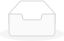# Electrical Machines

Electrical machines subject is the heart of Electrical engineering. electrical machinery conducts an exhaustive coverage of transformers, d.c. Machines and 3-phase synchronous and induction machines. it highlights the fact that all electrical machines function on similar fundamental doctrines. Average weightage of this subject in GATE examination is 08 to 10 percentage.

#### ₹ 149 / ₹ 999

Electrical machines subject is the heart of Electrical engineering. electrical machinery conducts an exhaustive coverage of transformers, d.c. Machines and 3-phase synchronous and induction machines. it highlights the fact that all electrical machines function on similar fundamental doctrines.

It opens up with Basics of Network and Power system. Chapters like Objectives of transmission lines,Power flow equation and Power factor compensation method are divided in individual video lectures, covering each topic step wise.

Average weightage of this subject in GATE examination is 8 to 10%Learn through Expert facultiesUser Friendly interface

Chapter 2

### Transformers

2.1 Introduction

2.2 Transformer Construction

2.3 Principle of Transformer Action

2.4 Equivalent Circuit of A Transformer

2.5 Open – Circuited and Short – Circuit Tests

2.6 Voltage Regulation of A Transformer

2.7 Transformer Losses

2.8 Transformer Efficiency

2.9 Transformers Tests

2.10 Auto-Transformers

2.11 Three Phase Transformer

2.12 Gate Questions

Chapter 3

### Basic Concept of Rotating Machines

3.1 - Introduction

3.2 - Flux Density Waveform & Induced EMF Equation

3.3 - Three Phase Machine & Distributed Winding and Short Pitch

3.4 - Numerical Questions

3.5 - Slot Harmonics

Chapter 5

### Three Phase Induction Motor

Concept of Rotating Magnetic Field & Principle of Operation

Equivalent Circuit & Power flow

Torque Slip Characteristics

Stable & Unstable Region of Operation

No load Test & Blocked Rotor Test

Starting of Three Phase Induction Motor

Speed Control Of Three Phase Induction Motor

Logging & Crow ling

Single Phase Induction Motor

8 videos

Chapter 6

### Synchronous Machines

Introduction

Circuit Model of Synchronous Machine

Characteristics of Synchronous Machine

Short-Circuit Ratio (SCR)

Potier Method

Operating Characteristics

Power Flow (Transfer) Equations

Capability Curve of Synchronous Generator

Salient-Pole Synchronous Machine Two-Reaction Model

Power-Angle Characteristic

Determination of Xd And Xq –Slip Test

Parallel Operation of Synchronous Generators

Hunting in Synchronous Machines

Damper Winding

This Week

This Month

Later

This Week

This Month

Later

E-Book

### Reviews